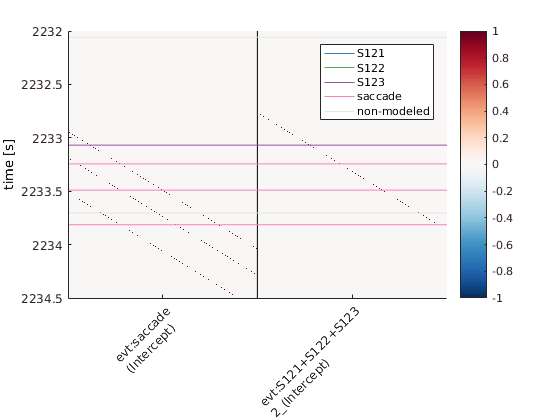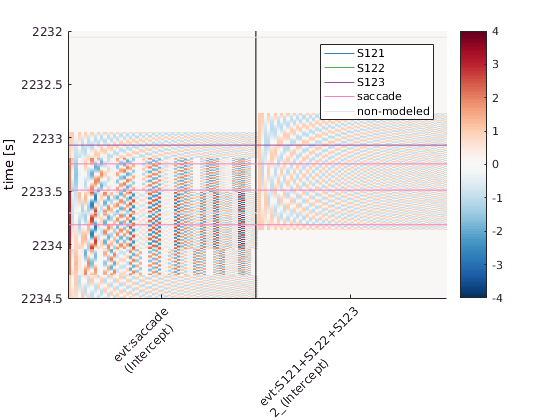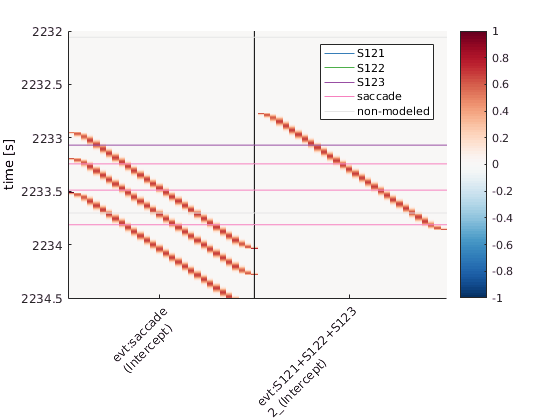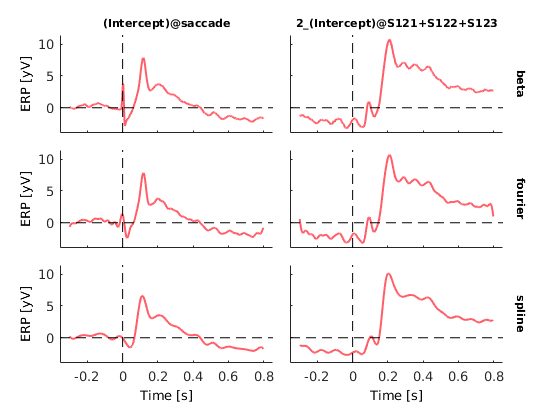init_unfold
Starting unfold toolbox. Adding subfolders and other toolboxes to path... Done.

# Introduction

Subjects are exploring a face stimulus. Using an eyetracker and the EEG-Eye toolbox we add markers of saccade onsets into our EEG-Data. The data were cleaned for eye-movement related artefacts using ICA.
In this tutorial we try to disentangle stimulus related ERPs and microsaccade related ERPs.
Find the data at: https://osf.io/wbz7x/
EEG = struct with fields:
setname: 'face_saccades_opendata' filename: 'face_saccades_opendata_fig10.set' filepath: 'C:\Users\behinger\Desktop' subject: '' group: '' condition: '' session: [] comments: 'Data to re-create Figure 10 in Ehinger & Dimigen, 2018, BioRxiv. Please do not use this data for other purposes without asking first (olaf.dimigen@hu-berlin.de)' nbchan: 40 trials: 1 pnts: 2070402 srate: 500 xmin: 0 xmax: 4.1408e+03 times: [1×2070402 double] data: [40×2070402 single] icaact: [] icawinv: [] icasphere: [] icaweights: [] icachansind: [] chanlocs: [1×40 struct] urchanlocs: [] chaninfo: [1×1 struct] ref: 'averef' event: [1×7661 struct] urevent: [1×7661 struct] eventdescription: {'' '' '' '' '' '' '' '' '' '' '' '' '' ''} epoch: [] epochdescription: {} reject: [1×1 struct] stats: [1×1 struct] specdata: [] specicaact: [] splinefile: '' icasplinefile: '' dipfit: [] history: '↵EEG.setname='MSAFF_01';↵EEG = eeg_checkset( EEG );↵EEG = eeg_checkset( EEG );↵EEG.etc.eeglabvers = '14.1.2'; % this tracks which version of EEGLAB is being used, you may ignore it↵EEG = pop_loadset('filename','face_saccades_opendata.set','filepath','M:\\Dropbox\\shared folders_main\\deconv\\figures\\toolbox_paper\\redo_figure10_to_match_opendata\\');↵EEG = eeg_checkset( EEG );↵pop_eegplot( EEG, 1, 1, 1);↵EEG = pop_select( EEG,'nochannel',{'L-Dia-X-(px)' 'L-Dia-Y-(px)' 'R-Dia-X-(px)' 'R-Dia-Y-(px)'});↵EEG = eeg_checkset( EEG );↵EEG = pop_saveset( EEG, 'filename','face_saccades_opendata_fig10.set','filepath','M:\\Dropbox\\shared folders_main\\deconv\\figures\\toolbox_paper\\redo_figure10_to_match_opendata\\');↵EEG = eeg_checkset( EEG );↵EEG = pop_select( EEG,'nochannel',{'L-GAZE-X-(px)' 'L-GAZE-Y-(px)' 'R-GAZE-X-(px)' 'R-GAZE-Y-(px)'});↵EEG = eeg_checkset( EEG );↵EEG.etc.eeglabvers = '14.1.1'; % this tracks which version of EEGLAB is being used, you may ignore it' saved: 'justloaded' etc: [1×1 struct] datfile: []
EEG = eeg_checkset(EEG);
% A simple threshold function taken from ERPLAB
not_eyechan = cellfun(@(x)~strcmpi(x,'eye'),{EEG.chanlocs.type});
winrej = uf_continuousArtifactDetect(EEG,'amplitudeThreshold',250,'channels',not_eyechan);
WARNING: boundary events were not found. So, basicrap.m will be applied over the full range of data. Please wait. This might take several seconds... 58 segments were marked. A total of 133034 samples (6.43 percent of data) was marked as bad.
% We remove very noisy data segments (>250mV) from the designmatrix

# Defining the design

cfgDesign = [];
% We use intercept-only formulas because we are only interested in the overlap for now
cfgDesign.formula = {'y ~ 1','y~1'};
EEG = uf_designmat(EEG,cfgDesign);
Multiple events with separate model-formula detected Modeling 2871 event(s) of [saccade] using formula: y~1 Modeling 479 event(s) of [stimonset] using formula: y~1

# Timeshift

In order to compensate for the inevitable linear overlap between ERPs, we want to use timeshifting / prepare the designmatrix for the deconvolution step. In this step we generate for each column of the designmatrix timeshifted (by t = 1, t=2, t=3 ...) and append them to the designmatrix.
cfgTimexpand = [];
cfgTimexpand.timelimits = [-.3,0.8];
cfgTimexpand.method = 'stick';
EEG1 = uf_timeexpandDesignmat(EEG,cfgTimexpand);
uf_timeexpandDesignmat(): Timeexpanding the designmatrix... ...done
cfgTimexpand.method = 'fourier';
cfgTimexpand.timeshiftparam = 20; % use frequencies up to (dF = 1/T): 1/(1.1s) *20 ~ 18Hz
% effective parameters are here twice as high because each frequ. is defined by cos+sin
EEG2 = uf_timeexpandDesignmat(EEG,cfgTimexpand);
uf_timeexpandDesignmat(): Timeexpanding the designmatrix... frequency resolution 1/T = 0.91 Hz rounding timeexpandparam from even 60 to odd 59 Fourier basisfunctions: using DC + the lower frequencies up to 26.36 Hz (approximation) Convolving and adding row 1 from 2 to designmatrix Convolving and adding row 2 from 2 to designmatrix ...done
cfgTimexpand.method = 'splines';
cfgTimexpand.timeshiftparam = 40; % use 40 splines over the 1.1s epoch (equivalent number of effective parameters as fourier)
EEG3 = uf_timeexpandDesignmat(EEG,cfgTimexpand);
uf_timeexpandDesignmat(): Timeexpanding the designmatrix... Convolving and adding row 1 from 2 to designmatrix Convolving and adding row 2 from 2 to designmatrix ...done
uf_plotDesignmat(EEG1,'timeexpand',1)
ylim([2232 2234.5])uf_plotDesignmat(EEG2,'timeexpand',1)
ylim([2232 2234.5])uf_plotDesignmat(EEG3,'timeexpand',1)
ylim([2232 2234.5])# Cleaning of data

EEG1 = uf_continuousArtifactExclude(EEG1,struct('winrej',winrej));
Portions of data removed split up by each event in EEG.event Type: S 99 modelled eventtime: 1054.90s rejected eventtime: 16.33s percent removed: 1.5% Type: S100 modelled eventtime: 1.10s rejected eventtime: 1.10s percent removed: 100.0% Type: S200 modelled eventtime: 1.10s rejected eventtime: 0.00s percent removed: 0.0% Type: buttonpress modelled eventtime: 526.90s rejected eventtime: 7.25s percent removed: 1.4% Type: fixation modelled eventtime: 862.05s rejected eventtime: 11.27s percent removed: 1.3% Type: saccade modelled eventtime: 861.57s rejected eventtime: 11.27s percent removed: 1.3% Type: stimonset modelled eventtime: 526.90s rejected eventtime: 8.34s percent removed: 1.6% Removing 6.43% of rows from design matrix (filling them with zeros)
EEG2 = uf_continuousArtifactExclude(EEG2,struct('winrej',winrej));
Portions of data removed split up by each event in EEG.event Type: S 99 modelled eventtime: 1054.90s rejected eventtime: 16.33s percent removed: 1.5% Type: S100 modelled eventtime: 1.10s rejected eventtime: 1.10s percent removed: 100.0% Type: S200 modelled eventtime: 1.10s rejected eventtime: 0.00s percent removed: 0.0% Type: buttonpress modelled eventtime: 526.90s rejected eventtime: 7.25s percent removed: 1.4% Type: fixation modelled eventtime: 862.05s rejected eventtime: 11.27s percent removed: 1.3% Type: saccade modelled eventtime: 861.57s rejected eventtime: 11.27s percent removed: 1.3% Type: stimonset modelled eventtime: 526.90s rejected eventtime: 8.34s percent removed: 1.6% Removing 6.43% of rows from design matrix (filling them with zeros)
EEG3 = uf_continuousArtifactExclude(EEG3,struct('winrej',winrej));
Portions of data removed split up by each event in EEG.event Type: S 99 modelled eventtime: 1054.90s rejected eventtime: 16.33s percent removed: 1.5% Type: S100 modelled eventtime: 1.10s rejected eventtime: 1.10s percent removed: 100.0% Type: S200 modelled eventtime: 1.10s rejected eventtime: 0.00s percent removed: 0.0% Type: buttonpress modelled eventtime: 526.90s rejected eventtime: 7.25s percent removed: 1.4% Type: fixation modelled eventtime: 862.05s rejected eventtime: 11.27s percent removed: 1.3% Type: saccade modelled eventtime: 861.57s rejected eventtime: 11.27s percent removed: 1.3% Type: stimonset modelled eventtime: 526.90s rejected eventtime: 8.34s percent removed: 1.6% Removing

# Fit the deconvolution model

EEG1= uf_glmfit(EEG1,'channel',);
uf_glmfit(): Fitting deconvolution model...solving the equation system solving electrode 32 (of 1 electrodes in total)... 16 iterations, took 0.2s LMfit finished
EEG2= uf_glmfit(EEG2,'channel',);
uf_glmfit(): Fitting deconvolution model...solving the equation system solving electrode 32 (of 1 electrodes in total)... 14 iterations, took 1.7s LMfit finished
EEG3= uf_glmfit(EEG3,'channel',);
uf_glmfit(): Fitting deconvolution model...solving the equation system solving electrode 32 (of 1 electrodes in total)... 35 iterations, took 0.5s LMfit finished

# Plot the results

We choose a single electrode and plot all effects.
ufresult1= uf_condense(EEG1);
ufresult2= uf_condense(EEG2);
ufresult3= uf_condense(EEG3);
% Our plotting function allows for arbitrary additional fields
% as long as they have the same size as unfold.beta
ufresult = ufresult1;
ufresult.fourier = ufresult2.beta;
ufresult.spline= ufresult3.beta;
display(ufresult)
ufresult =
unfold: [1×1 struct] beta: [48×550×2 double] times: [1×550 double] chanlocs: [1×48 struct] param: [1×2 struct] fourier: [48×550×2 double] spline: [48×550×2 double]
uf_plotParam(ufresult,'channel',32);
Warning: A value of class "com.mathworks.mde.cmdwin.XCmdWndView" was indexed with no subscripts specified. Currently the result of this operation is the indexed value itself, but in a future release, it will be an error.
Warning: A value of class "com.mathworks.mde.cmdwin.XCmdWndView" was indexed with no subscripts specified. Currently the result of this operation is the indexed value itself, but in a future release, it will be an error.
plotting all parametersThe first model has 550 parameter per predictor, the second and third one only 40 per predictor. This can show up in memory performance for large models. But due to the great sparseness of the first model (the stick-functions), it is actually often the fastest one..